# Chapter 2a: acid-base reactions

In this module we will review one of the main types of reaction of chemistry. Reactions can indeed be classified in 3 major categories:

• Acid-base reactions
• Redox (reduction and oxidation) reactions
• Solubility reactions (dissolution and precipitation)

The two last reaction types are seen in other sections of our lessons. We will here focus on acid-base reactions. The first step will be to introduce the definitions of acid and basic compounds and the notion of acidity. We will see next the strength of different acids and bases and explain how to follow experimentally the neutralisation of an acid by a base.

Definitions

A proton is a Hydrogen (H) atom that lost its electron (e). Consequently, a proton consists only in one nucleus, which is positively charged. The proton is an ion: a charged molecule. We have here to consider that the nucleus of an atom is only a very small fraction of the volume of an atom (radius of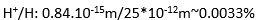). Because of its small size, a proton can diffuse in everything and move through any material until its neutralization.

Several definitions were given to an acid and a base. Arrhenius proposed

• An acid is a donator of protons
• A base is a donator of OH

This definition works well for several compounds, for example: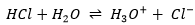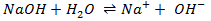However, some basic compounds do not possess any OH group in them and can still neutralize acids. For example NH3 can react with H+ but cannot free any OH-. A tentative of explanation was to introduce NH4OH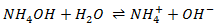but this compound does simply not exist.

Brønsted and Lowry proposed another theory:

• An acid is a donator of protons
• A base is an captor of protons

When a base reacts with an acid, they form respectively their conjugate acid and conjugate baseConsidering any acid HA, the equation can be written: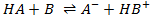HA loses a proton to form its conjugate base A. The base B receives the proton to form its conjugate acid HB+.

An interesting point of this theory is that the acidity of a compound depends on the reaction in which it intervenes. It allows to some compounds to be both an acid and a base. For example, H2O can donate or receive protons.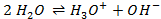Such compound is called amphoteric. In water, there is thus both acid and base in water. However, when we drink or put a hand into water, we don’t feel those substances (note that the water that we usually use in our everyday tasks contains ions, modifying its taste and slightly its acidity). As explained earlier, an acid attacks deeply into materials. Bases on the other hand affect surfaces by removing their protons. So why is nothing happening? The reason why is because the three substance (H2O, H3O+ and OH) are in equilibrium. The reaction just above goes in both directions, as it is shown by the double arrow. However, the reaction doesn’t go in both directions at the same speed. The equilibrium constant for the reaction going from left to right is Kw=10-14mol2l-2=[H3O+][OH]. For the other direction, the constant is K=1/Kw=1014mol-2l2. That means that the equilibrium is highly oriented towards the left. It is not frequent that a molecule of H2O autoprotolyses and when it occurs, the inverse reaction is very fast.

From Kw, we can determine the concentration of protons (or H3O+) in water.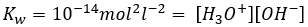Because H3O+ and OH are produced at the same rate, their concentration is equal: [H3O+]=[OH]. Then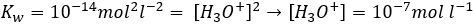In pure water, the concentration of protons is thus 10-7M (M=mol/l) at any moment. If an acid is put into water, the amount of protons in solution will increase. Inversely, if a base is put in water, the amount of protons decreases. The acidity of a solution is thus measured by the concentration of protons in the solution. For more comfort, the scale, called potential Hydrogen or pH, is minus the logarithm of the concentration of protons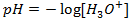and goes from 0 to 14 in aqueous solutions. At pH=0, the concentration of protons in solution is 1M. At pH=14, barely all the protons are removed from the solution by the base. The pH is not infinite because there is always a few protons remaining in the solution, due to the equilibrium. pH=7 is the neutral pH and is the pH of pure water. Most of the living species are adapted to this neutral pH. Some others have adapted to basic or acidic conditions to avoid predation or concurrence for resources.

We can also talk about pOH for bases with pOH=-log [OH]. pOH is however generally not used. For basic aqueous solution, it is easier to refer at pH=14-pOH=14+log[OH].

Lewis acids and bases

The same year, Gilbert Newton Lewis proposed an alternative, and broader, definition for acids and bases: A Lewis base is defined as a compound that can donate an electron pair to a Lewis acid, a compound that can accept an electron pair. Considering the same notation as above,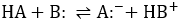The two dots in this notation represents the pair of electron that the Lewis base B and the conjugate base A are carrying. The proton is a Lewis acid, accepting pairs of electrons. With such a definition, the acids are no more limited to substances carrying hydrogen atoms. For example BF3 is a Lewis acid as the bore can accept a pair of electron.

Dissociation of H and OH

It is to be noted that in some occasions, H is not dissociated as a proton. When the liaison between two atoms is broken, the pair of electrons remains with atom of greater electronegativity (χ).

Examples:

H-Cl:                χCl=3.16                                 χH=2.2

In this case, and as expected, the pair of electrons remains on the chloride atom because its electronegativity is larger than the one of the Hydrogen.

Na-H:              χNa=0.93                                χH=2.2

The Sodium hydride is one of the few exceptions where the Hydrogen atom takes the pair of electrons. Indeed, its electronegativity is very low in comparison with H. This molecule splits in Na+ and H.

If we look now to the liaison OH:

χO=3.44                                 χH=2.2

This group, typical in the basic compounds, can break to free a proton. A molecule carrying a OH group may then be acidic or basic depending on the atom connected to the oxygen.

In NaOH for example, the electronegativity of Na (χNa=0.93) is smaller than the one of the hydrogen (χH=2.2), by far. As a result, it is the bond between Na and O that breaks. As the O is already negatively charged, the O-H bond won’t split to give O2- and H+.

On the contrary, in HClO (Cl-O-H), the electronegativity difference between Cl-O is larger than the one between O-H. As a result, the hypochlorous acid splits in ClO and H+.

To resume, the acidity of a substance depends on the reaction in which it takes part, and the presence of a H or a OH group in the substance does not mean that it is an acid or a base, and vice versa the fact that a substance is acidic or basic does not mean that this substance carries a H or OH group.

Measure of pH

Different methods exist to measure or to give an idea of the pH of a solution.

pH indicator

When a few droplets of pH indicator are added to a solution, the pH indicator gives the solution a colour depending on the acidity of the solution. Into a given area of pH the solution will be of a certain colour while the colour is different into another area of pH. Those area are not specifically 0-7 and 7-14 and depend on the pH indicator used. The change of colour is due to interactions between protons and the molecules of the pH indicator.

For example, bromocresol green is yellow in its acidic form and blue in its basic form. There is a transition area of pH for the bromocresol green between the pH from 3,8 to 5,4 where its colour is green, the colour of “its neutral form” (in fact it is a mix of the acidic and the basic forms). The structure of bromocresol green is shown is the Figure 1. The colour of the solution does not vary sensibly in the same area of pH but only at the limits between two area. It is explained by the fact that only a few drops are enough to obtain a visible colour. Moreover, as there are interactions between the pH indicator and the protons, the pH of the solution is affected by the presence of the pH indicator.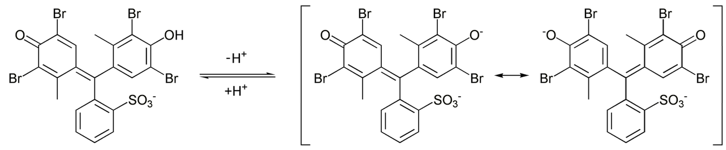Figure 1: Structure of the bromocresol green in its acidic (left) and basic (middle and right) form. There are two resonance structures of the basic compound. Resonance will be seen in further chapters (organic chemistry)

Note that at pH=5.5 for example, this indicator is in its basic form even if the solution is acidic. pH indicators are thus useful to have an idea of the acidity of the solution. However, a lot of pH indicators exist and are easy to use.

pH paper

The pH paper is a paper containing several pH indicators.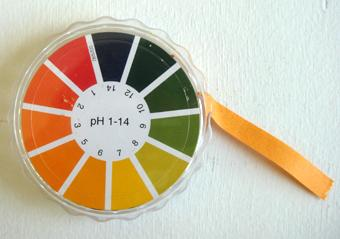Initially jellow, its colour varies with the pH of the solution, from deep red for acids to deep blue for bases. Usually one droplet of the solution is dropped on the pH paper, giving it its colour. One can next compare the colour of the pH paper with a scale on the box of the pH paper to determine the pH of the solution.

pH meter

This device determines the concentration of protons in solution thanks to an electrode plunged in the solution. It is more accurate than the two other methods but may need calibration. Its functioning will be seen later.